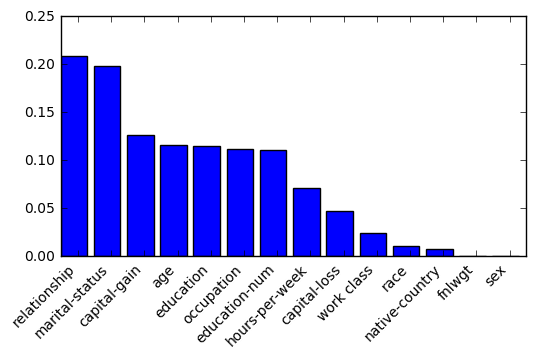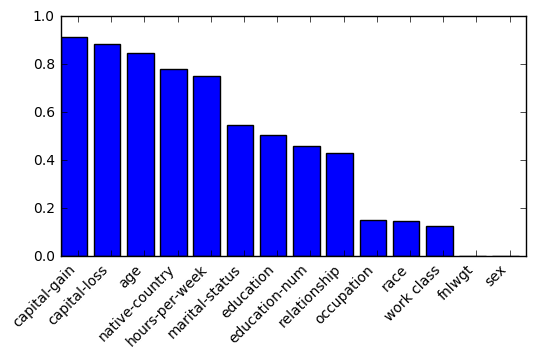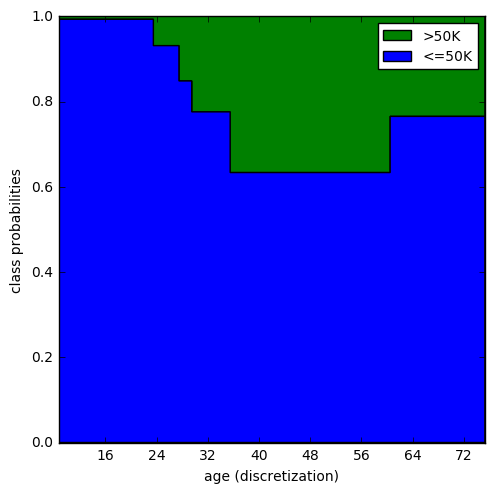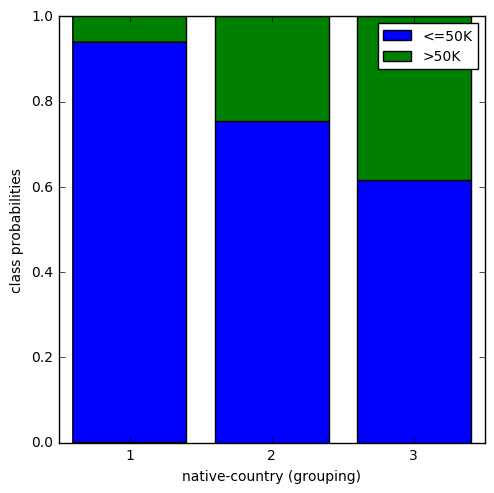# Edge ML tutorial¶

### Step #1: Automaticaly build robust classifiers¶

#1.1 Data preparation

In :
import os
import pandas as pd
import numpy as np
from lib.edgeML import *                                   # let's import the edgeML wrapper

from sklearn.cross_validation import train_test_split
import matplotlib.pyplot as plt


This example is based on the 'adult' dataset available at https://archive.ics.uci.edu/ml/machine-learning-databases/adult/. The explicative variables describe some people, and the target variable indicates the level of the salaries of each person (more or less than 50 K dollars by year).

In :
X = pd.read_csv("./data/adult.txt", sep='\t')

Out:
age work class fnlwgt education education-num marital-status occupation relationship race sex capital-gain capital-loss hours-per-week native-country salary
0 50 Self-emp-not-inc 83311 Bachelors 13 Married-civ-spouse Exec-managerial Husband White Male 0 0 13 United-States <=50K
1 38 Private 215646 HS-grad 9 Divorced Handlers-cleaners Not-in-family White Male 0 0 40 United-States <=50K

Let's prepare the train and test sets

In :
y = X['salary']
X = X.drop('salary', axis=1)

X_train, X_test, y_train, y_test = train_test_split(X, y, test_size=0.30, random_state=42)


#1.2 Fit a classifier

Now, a classifier is instantiated by edgeML

In :
classifier = edgeML()


The edgeML's wrapper need to handle temporary files stored in an automaticaly created folder. The constructor of edgeML takes two parameters as input: i) the first one is the path where the folder is created; ii) the second parameter is the name of the folder. Let us fit the clasifier !

In :
%time classifier.fit(X_train, y_train)

CPU times: user 400 ms, sys: 20 ms, total: 420 ms
Wall time: 14.4 s


edgeML is an autonomous Machine Learning algorithme which optimaly find the best model. There is no cross validation, there is no user parameter to be tunned. The outcome classifier is robust: overfitting is purely avoided.

#1.3 Evaluation

At this step, the classifier is already evaluated on the train set.

In :
print("Accuracy :\t\t"+str(classifier.accuracy_Train))
print("average AUC :\t\t"+str(classifier.avg_AUC_Train))
print("classes :\t\t"+str(classifier.labels))
print("AUC by class :\t\t"+str(classifier.AUC_Train))

Accuracy :		0.860828
average AUC :		0.920488
classes :		['<=50K', '>50K']
AUC by class :		[0.920493, 0.920483]


Now, the learned classifier is evaluated on the test set

In :
classifier.score(X_test,y_test)

Out:
0.858825
In :
print("Accuracy (test) :\t\t"+str(classifier.accuracy_Test))
print("average AUC (test) :\t\t"+str(classifier.avg_AUC_Test))
print("classes :\t\t\t"+str(classifier.labels))
print("AUC by class (test) :\t\t"+str(classifier.AUC_Test))

Accuracy (test) :		0.858825
average AUC (test) :		0.917346
classes :			['<=50K', '>50K']
AUC by class (test) :		[0.917358, 0.917334]


As usual, the AUCs on the test and train sets are very close. Now you can easily plot the ROC curves as follows.

In :
classifier.plotROC()


#1.4 Features importance

edgeML provides two different ranking of the variables: i) the 'univariate feature importance' which is based on the compression gain of dicretization and grouping models; ii) the 'multivariate feature importance' which corresponds to the weights of variables within the trained ensemble Bayesian classifier.

In :
classifier.features_importance_univar()

Out:
variable importance
10 relationship 0.208405
8 marital-status 0.197436
3 capital-gain 0.125984
0 age 0.115955
7 education 0.114394
9 occupation 0.111572
2 education-num 0.110629
5 hours-per-week 0.071272
4 capital-loss 0.047001
6 work class 0.023553
11 race 0.010285
13 native-country 0.007387
1 fnlwgt 0.000000
12 sex 0.000000
In :
classifier.plotImportanceUnivar()IMPORTANT NOTICE: the variables which have a zero univariate weigth must be removed from the dataset (ex: fnlwgt, sex). These variables correspond to random class values over the numerical domains. edgeML provides a very usefull way of removing uninformative variables from a dataset, even if you train another classifier (random forest, GBM ... etc.). The useless variables can be displayed as follows:

In :
classifier.uselessVar()

Out:
drop variables
2 fnlwgt
3 sex

Now, let us take a look at the multivariate feature importance.

In :
classifier.features_importance()

Out:
variable importance
3 capital-gain 0.912090
4 capital-loss 0.883296
0 age 0.844966
13 native-country 0.779378
5 hours-per-week 0.748304
8 marital-status 0.545503
7 education 0.504341
2 education-num 0.456347
10 relationship 0.427937
9 occupation 0.151777
11 race 0.147034
6 work class 0.126181
1 fnlwgt 0.000000
12 sex 0.000000
In :
classifier.plotImportanceMultivar()Contrary to the previous ranking, the multivariate feature importance takes into account the interactions between variables. For instance, the 'native-country' variable has a relatively important multivariate weight, while its univariate weight is small (see previous plot).

Now, let us take a look at the univariate discretization and grouping models. The following command line plots the conditionnal distribution of class values over the numerical domaine of the variable 'age'. The probablity to have a salary greater than 50 k\$ is maximum when the age is between 35 years and 60 years.

In :
classifier.plotDiscretization('age')In the same way, this command line allows you to plot the conditional distribution of class value for categorical variables. The composition of each group is printed bellow the chart.

In :
classifier.plotDiscretization('native-country')Groupe 1 : Vietnam, Jamaica, Mexico, Puerto-Rico, El-Salvador, Peru, Columbia, Haiti, Dominican-Republic, Laos, Ecuador, Trinadad&Tobago, Outlying-US(Guam-USVI-etc), Nicaragua, Guatemala, Honduras, Thailand, Holand-Netherlands, Missing

Groupe 2 : United-States, China, Philippines, ?, Cuba, Portugal, Ireland, South, Poland, Greece, Hungary, Scotland

Groupe 3 : France, India, Germany, Hong, Taiwan, Canada, Italy, Iran, Yugoslavia, Japan, Cambodia, England



In the end, the grouping and disctretization models are fully discribed by the dictionnary 'dico_partition'

In :
classifier.dico_partition['age']

Out:
{'bounds': [23.5, 27.5, 29.5, 35.5, 60.5],
'nbPart': 6,
'proba': [[0.992887, 0.007113],
[0.931034, 0.068966],
[0.848229, 0.151771],
[0.775685, 0.224315],
[0.633293, 0.366707],
[0.765064, 0.234936]],
'varType': 'numerical'}

### Step #2: Make predictions¶

In :
Prev = classifier.predict(X_test)

Out:
most_probable_label
0 <=50K
1 <=50K
2 >50K
3 <=50K
4 >50K
In :
Prev = classifier.predict_proba(X_test)

Out:
P(<=50K|X) P(>50K|X)
0 0.978884 0.021116
1 0.603115 0.396885
2 0.373401 0.626599
3 0.616886 0.383114
4 0.474486 0.525514

### Step #3: Recode a data set¶

Univariate discretization and grouping modeles can be exploited to recode a dataset. Then, you can train another classifier by using the recoded dataset. There are two advantages to recode data: i) a categorical variable (with a large number of levels) is recoded into numerical variables which estimate the distribution of the class values; ii) the discretization and grouping models are regularized, so the recoded data reduces the risk of overfitting regarless of the learned classifier.

In :
newDataSet = classifier.transform(X_test)

Out:
Proba_age_>50K Proba_work class_>50K Proba_fnlwgt_>50K Proba_education_>50K Proba_education-num_>50K Proba_marital-status_>50K Proba_occupation_>50K Proba_relationship_>50K Proba_race_>50K Proba_sex_>50K Proba_capital-gain_>50K Proba_capital-loss_>50K Proba_hours-per-week_>50K Proba_native-country_>50K
0 0.366707 0.220415 0.239919 0.158031 0.158031 0.099559 0.122677 0.064503 0.255623 0.239909 0.202751 0.226406 0.067195 0.244717
1 0.366707 0.279204 0.239919 0.158031 0.158031 0.446669 0.465448 0.451901 0.255623 0.239909 0.202751 0.226406 0.205111 0.244717
2 0.151771 0.220415 0.239919 0.420896 0.420896 0.446669 0.465448 0.451901 0.118189 0.239909 0.202751 0.226406 0.431023 0.244717
3 0.366707 0.220415 0.239919 0.744361 0.623181 0.045725 0.465448 0.100913 0.255623 0.239909 0.202751 0.226406 0.205111 0.244717
4 0.366707 0.220415 0.239919 0.555835 0.623181 0.099559 0.465448 0.100913 0.255623 0.239909 0.202751 0.226406 0.349341 0.244717

edgeML provides several ways of encoding data. For more delails, see the help page by typing 'edgeML -?' in a terminal, and see the Step #4 of this tutorial. The following command line links up the 'fit' and the 'transform' steps:

In :
newDataSet = classifier.fit_transform(X_train, y_train)

Out:
Proba_age_>50K Proba_work class_>50K Proba_fnlwgt_>50K Proba_education_>50K Proba_education-num_>50K Proba_marital-status_>50K Proba_occupation_>50K Proba_relationship_>50K Proba_race_>50K Proba_sex_>50K Proba_capital-gain_>50K Proba_capital-loss_>50K Proba_hours-per-week_>50K Proba_native-country_>50K
0 0.224315 0.220415 0.239919 0.420896 0.420896 0.045725 0.465448 0.100913 0.255623 0.239909 0.202751 0.226406 0.205111 0.244717
1 0.007113 0.220415 0.239919 0.158031 0.158031 0.045725 0.224975 0.015691 0.255623 0.239909 0.202751 0.226406 0.067195 0.060697
2 0.224315 0.100529 0.239919 0.158031 0.158031 0.099559 0.122677 0.100913 0.255623 0.239909 0.202751 0.226406 0.205111 0.244717
3 0.366707 0.372230 0.239919 0.555835 0.623181 0.099559 0.122677 0.064503 0.255623 0.239909 0.202751 0.226406 0.205111 0.244717
4 0.366707 0.220415 0.239919 0.744361 0.623181 0.446669 0.465448 0.451901 0.255623 0.239909 1.000000 0.226406 0.431023 0.244717

### Step #4: Set options¶

Several options of edgeML can be set by using a dictionnary. Here are the default values of the parameters:

In :
params = {
'lessVar': False,
'nb_jobs': -1,
'lambda': 1,
'inplace': True,
'transform_var': 'all',
'transform_type': 'proba'
}


'lessVar' is an option which tries to remove variables in order to improve the quality of the classifier. This option is usefull when the dataset includes a large number of variables (The possible values of this parameter are 'True' or 'False'). The selected variables can be displayed by the commande line 'classifier.selectedVar()'.

'nb_jobs' is a parameter which indicates the number of cores that edgeML use. The value '-1' indicates that all the cores of your computer will be exploited.

'lambda' is a float parameter which allows you to train over-fitted models which include more intervals and groups than the optimal models. The value of this parameter (in [0,1]) is related to the decreasing of the compression gains. For instance, 'lambda : 0.9' reaches to models with 10% lower compression gains. In some cases, the over-fitting option may improve the classifier, but the robustness is not ensured anymore.

'inplace' is a parameter which indicates if the original columns of a recoded data set are deleted ('inplace : True') are keept ('inplace : False').

'transform_var' is a parameter which indicates which type of variables must be modified when a data set is recoded (all, categorical, numerical)

'transform_type' indicates the way of recoding a data set. The possible values are the following: i) 'proba' to encode the conditional probabilities P(class|variable); ii) 'QI' to encode the quantity of information -log(P(class|variable)); iii) 'partition' to encode the group IDs and the interval IDs.

You can modifty the previously presented dictionnary, and set the new parameters as follows:

In :
classifier.set_params(**params)


The current parameters of a classifier can be displayed as follows:

In :
classifier.get_params()

Out:
{'inplace': True,
'lambda': 1,
'lessVar': False,
'nb_jobs': -1,
'transform_type': 'proba',
'transform_var': 'all'}

### Step #5: Evaluates the drift between the train and test sets¶

Sometimes, a concept drift occurs between the train set and data on which we make the predictions. edgeML is able to reliably measure the drift between two datasets (i.e. the difference of distribution for each variables). In the following example, we simulate a drift by removing pepole with less than 16 years of education from the test set.

In :
Train = pd.read_csv("./data/TRAIN_adult.txt", sep='\t')
Test = Test[Test['education-num'] > 15]


As previously, we build the train and test sets ...

In :
y_train = Train['salary']
X_train = Train.drop('salary', axis=1)
y_test = Test['salary']
X_test = Test.drop('salary', axis=1)


The drift between both datasets is evaluated as follows:

In :
classifier.driftEval(X_train,X_test)

Out:
Drift Level
education 0.715101
education-num 0.707609
occupation 0.19206
work class 0.0361472
age 0.0337004
relationship 0.0207277
marital-status 0.00502384
native-country 0.00416144
race 0.00133269
sex 0.00111337
capital-loss 0.000911458
fnlwgt 0
capital-gain 0
hours-per-week 0

In this example, two variables are detected as drifting significanty, namely 'education' and 'education-num'. These variables can be removed from the train set in order to reduce the risk of bad predictions on the test set.

### Step #6: Manage your models¶

A classifier can be exported as follows:

classifier.export('/home/edgeml/Desktop')

And you can import a previously learned classier as follows: